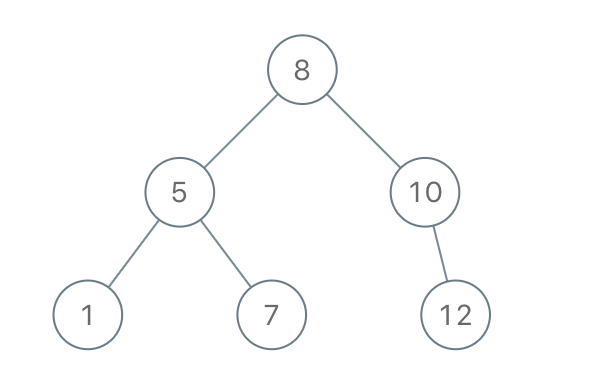# 1008. Construct Binary Search Tree from Preorder Traversal | LeetCode Solution

## 1008. Construct Binary Search Tree from Preorder Traversal | LeetCode Solution

Given an array of integers preorder, which represents the preorder traversal of a BST (i.e., binary search tree), construct the tree and return its root.

It is guaranteed that there is always possible to find a binary search tree with the given requirements for the given test cases.

binary search tree is a binary tree where for every node, any descendant of `Node.left` has a value strictly less than `Node.val`, and any descendant of `Node.right` has a value strictly greater than `Node.val`.

preorder traversal of a binary tree displays the value of the node first, then traverses `Node.left`, then traverses `Node.right`.

Example 1:```Input: preorder = [8,5,1,7,10,12]
Output: [8,5,10,1,7,null,12]
```

Example 2:

```Input: preorder = [1,3]
Output: [1,null,3]
```

Constraints:

• `1 <= preorder.length <= 100`
• `1 <= preorder[i] <= 1000`
• All the values of `preorder` are unique.

/**
* Definition for a binary tree node.
* struct TreeNode {
*     int val;
*     TreeNode *left;
*     TreeNode *right;
*     TreeNode() : val(0), left(nullptr), right(nullptr) {}
*     TreeNode(int x) : val(x), left(nullptr), right(nullptr) {}
*     TreeNode(int x, TreeNode *left, TreeNode *right) : val(x), left(left), right(right) {}
* };
*/
class Solution
{
public:
TreeNode *bstFromPreorder(vector<int> &v)
{
int n = v.size();
TreeNode *ans = new TreeNode(v);
TreeNode *curr = ans;
int i = 1;
stack<TreeNode *> st;
while (i < v.size())
{
if (curr->val > v[i])
{
curr->left = new TreeNode(v[i]);
st.push(curr);
curr = curr->left;
}
else
{
while (!st.empty() && st.top()->val < v[i])
{
curr = st.top();
st.pop();
}
curr->right = new TreeNode(v[i]);
curr = curr->right;
}
i++;
}
return ans;
}
};